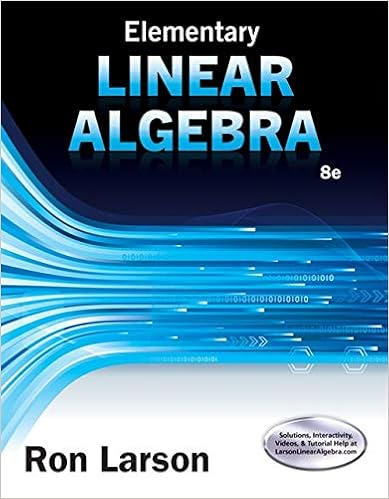# 1 experiment 2 theory a vector is a physical quantity

• Notes
• 4
• 50% (6) 3 out of 6 people found this document helpful

This preview shows page 1 - 3 out of 4 pages.

##### We have textbook solutions for you!
The document you are viewing contains questions related to this textbook.The document you are viewing contains questions related to this textbook.
Chapter 4 / Exercise 60
Elementary Linear Algebra
LarsonExpert Verified
- 1 -
##### We have textbook solutions for you!
The document you are viewing contains questions related to this textbook.The document you are viewing contains questions related to this textbook.
Chapter 4 / Exercise 60
Elementary Linear Algebra
LarsonExpert Verified
Experiment 2THEORYA vector is a physical quantity that has both magnitude and direction. Examples include force, weight, displacement, velocity and acceleration. On the other hand, a scalar is a physical quantity that has magnitude only, examples speed, mass, distance, temperature, population. A vector may be represented by a letter example with a bar on top indicating the direction, or by a bold letter, example AA vector A can be writtenas a sum of two vectorsAxandAy along the x and the y-axis respectively, as shown.Axand Ayare calledthe componentsof vector A and are given byAx= A cosα Ay= A sinα α (≤ 900)is the angle vector Amakes with the x-axis.In order to find the resultant vector Rof a system of vectors A, B, C, etc we follow these steps:a) Find the x and y components for each vector using the above equations. i.e find.:
y ....
•••